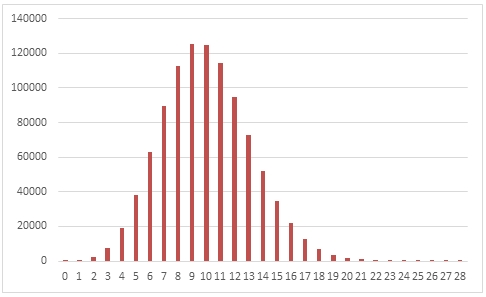# SQL Server random poisson distribution

RANDPOISSON

Updated: 31 March 2014

Use the table-valued function RANDPOISSON to generate a sequence of random integers from the Poisson distribution for a given @lambda.
Syntax
SELECT * FROM [wctMath].[wct].[RANDPOISSON](
<@Rows, int,>
,<@lambda, float,>)
Arguments
@Rows
the number of rows to generate. @Rows must be of the type int or of a type that implicitly converts to int.
@lamda
the lambda parameter to the distribution. @lambda must be of the type float or of a type that implicitly converts to float.
Return Types
RETURNS TABLE (
[Seq] [int] NULL,
[X] [int] NULL
)
Remarks
·         @lambda must be greater than zero.
·          If @lambda is NULL then @lambda is set to 1.
·         If @Rows is less than 1 then no rows are returned.
Examples
In this example we create a sequence 1,000,000 random numbers from a Poisson distribution with @lambda = 10, COUNT the results, paste them into Excel and graph them.
SELECT
X,
COUNT(*) as [COUNT]
FROM (
SELECT
X
FROM wct.RANDPOISSON(
1000000,    --@Rows
10          --@lambda
)
)n
GROUP BY
X
ORDER BY
1
This produces the following result.In this example we generate 1,000,000 random numbers from a Poisson distribution with @lambda of 4. We calculate the mean, standard deviation, skewness, and excess kurtosis from the resultant table and compare those values to the expected values for the distribution.
DECLARE @size as int = 1000000
DECLARE @lambda as float = 4
DECLARE @mean as float = @lambda
DECLARE @var as float = @lambda
DECLARE @stdev as float = SQRT(@lambda)
DECLARE @skew as float = 1/@stdev
DECLARE @kurt as float = 1/@mean

SELECT
stat,
[RANDPOISSON],
[EXPECTED]
FROM (
SELECT
x.*
FROM (
SELECT
AVG(cast(x as float)) as mean_POISSON,
STDEVP(x) as stdev_POISSON,
wct.SKEWNESS_P(x) as skew_POISSON,
wct.KURTOSIS_P(x) as kurt_POISSON
FROM
wct.RANDPOISSON(@size,@lambda)
)n
CROSS APPLY(
VALUES
('RANDPOISSON','avg', mean_POISSON),
('RANDPOISSON','stdev', stdev_POISSON),
('RANDPOISSON','skew', skew_POISSON),
('RANDPOISSON','kurt', kurt_POISSON),
('EXPECTED','avg',@mean),
('EXPECTED','stdev',@stdev),
('EXPECTED','skew',@skew),
('EXPECTED','kurt',@kurt)
)x(fn_name,stat,val_stat)
)d
PIVOT(sum(val_stat) FOR fn_name in([RANDPOISSON],[EXPECTED])) P

This produces the following result (your result will be different).

 stat RANDPOISSON EXPECTED avg 3.997972 4 kurt 0.241558748 0.25 skew 0.499471478 0.5 stdev 1.999604933 2

### SupportCopyright 2008-2023 Westclintech LLC         Privacy Policy        Terms of Service